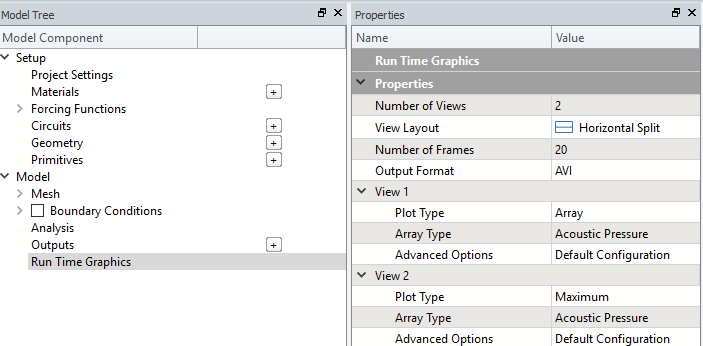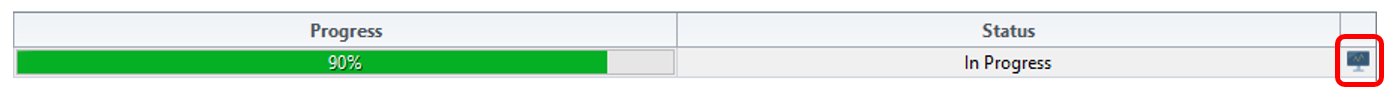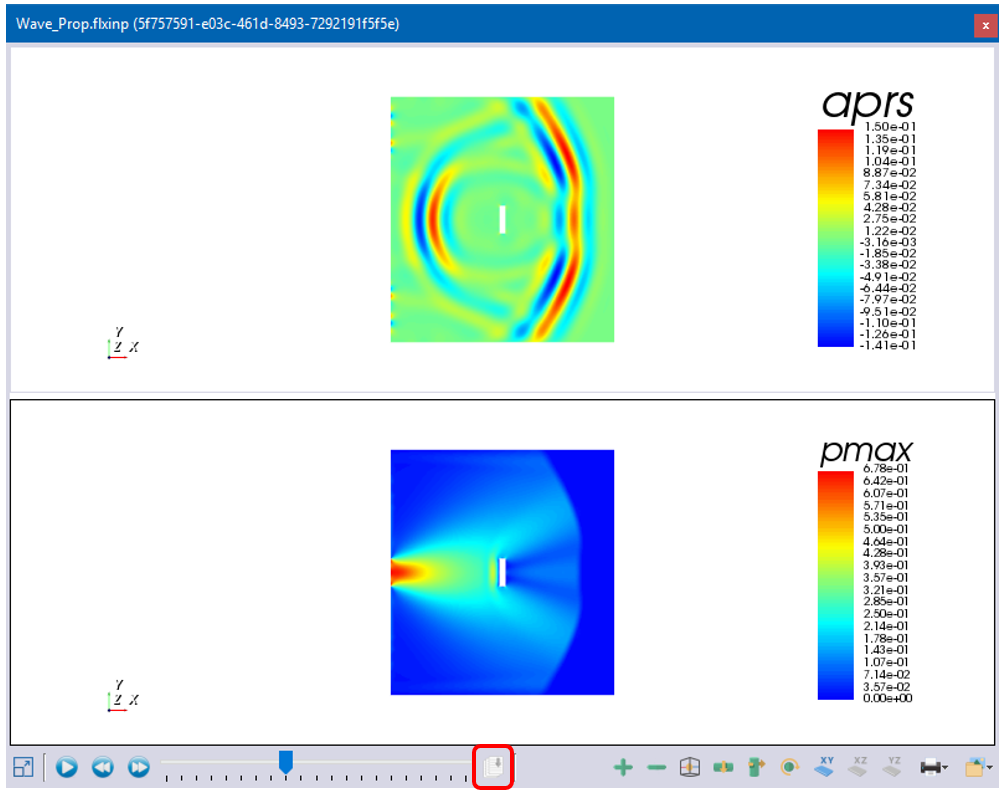# Cloud Runtime Graphics

Cloud Runtime graphics in OnScale allows users to instantaneously view data and plots, whilst the simulation processing on the cloud.

## Set Up

### Analyst Mode

There are three main steps to set up runtime graphics in Analyst Mode:

• Calculate necessary arrays of data
• Set up plotting
• Plot the calculated data arrays

Before any data can be plot, it must be calculated. This is done using the calc command. The following example calculates the acoustic pressure and the maximum pressure in the model. This calculation must be done before any process is carried out.

`/* Request calculation of additional arrayscalc    pres acoustic          /* Calculate acoustic pressure    max aprs none pmax     /* Calculate maximum pressure field    end`

The next step is to set up the plotting using the grph command. The following example sets the plotting layout as two plotting windows horizontally split. It also sets up the plot to be saved as a video with 10 frames per second. This part of the code also comes before the process stage.

`/* Set up plottinggrph    set imag avi 10        /* Set image capture for movie file generation    nvew 2 1               /* Set up 2 plotting windows in layout number 1    end`

The last step is to actually plot the data using the grph command and this occurs during the process. The following example plots the data arrays calculated above.

`/* Plot data arraysgrph     plot aprs               /* Plot calculated data array    plot pmax               /* Plot calculated data array    imag                    /* Snapshot of Graphics window for Movie generation    end`

### Designer Mode

Runtime graphics are set up in Designer Mode in the Run Time Graphics section of the Model Tree. The user must set the parameters required in the Properties window.## Accessing Graphics

Once the model is set up, it can then be run on the Cloud as usual. Runtime graphics can only be accessed once the job status is In Progress. They can be viewed via the button at the right hand side of the job or by right clicking the job and selecting Display Runtime Graphics.The runtime graphics window will plot each time it reaches a new frame. Once the job is finished, the user is able to download each frame via the button highlighted below and can cycle through the plot using the blue plot controls.The runtime graphics can be accessed from when they are created until 10 minutes after the simulation finishes.